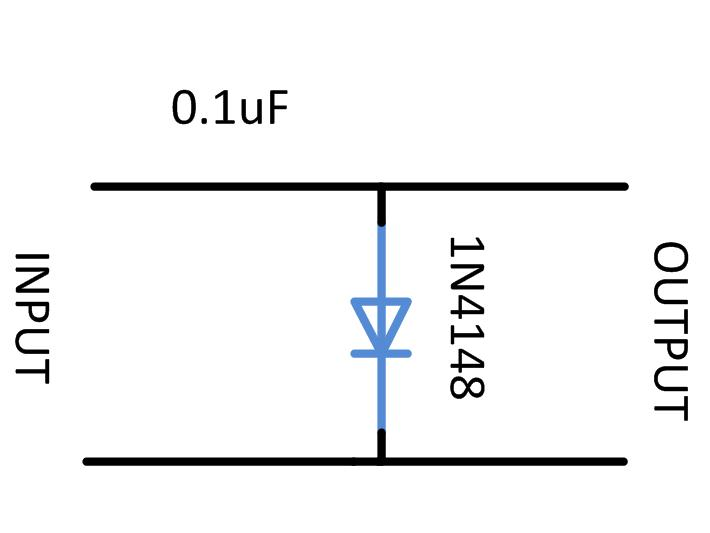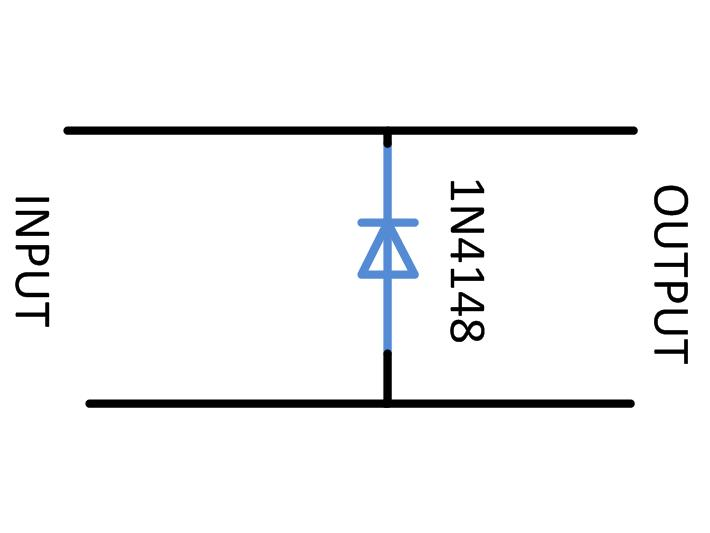﻿ Waveform Clipping: Positive & Negative Clipping Circuit Design

# DIY Circuit Design: Waveform Clipping

The electronic circuits like amplifiers, modulators etc. have a particular range at which they can accept the input signals. Any signals which have amplitude higher than this particular rage can cause distortion in the output of the circuit or may even cause damage to the circuit components itself. Since most of the devices works on single positive supply the input range will also be in the positive side. Since the natural signals like sine wave, audio signals etc. has both positive and negative cycles and varying amplitude they have to be modified in such a way the single supply electronic circuits can operate them.

Clipping of a wave is the common technique that is applied on the input signals to modify them so that they fall in the operating range of the circuits. The clipping is done by eliminating the portions of the waveform which are out of the input range of the circuit into which it needs to be applied. This article discusses the details of the clipping and the practical positive and negative clipping circuits.

DESCRIPTION:

The most commonly found circuit in which the clipping of a waveform is used is the rectifier circuit. In a rectifier circuit which produces positive output voltage from the sinusoidal input voltage, the negative half cycles of the sinusoidal waves are simply clipped away. In this case the method of clipping is called negative clipping and the method in which the entire positive half cycles are clipped away is called positive clipping. The positive clipping is used to obtain negative voltages with rectifiers in dual voltage power supplies. Simply using the technique of clipping the waveforms having both positive and negative half cycles can be clipped to either positive or negative voltage side only.

The following figures represent the clipping of a sine wave using both the positive and negative clipping devices.Fig. 1: Clipping of Sine Wave using both Positive and Negative Clipping Devices

The positive or negative clipping of a sine wave can be achieved by using a single diode only. Here the positive and negative clipping of a sine wave is demonstrated with the help of a Wien Bridge oscillator circuit which can generate a sine wave. The Wien Bridge is a circuit which can generate the pure sine wave with minimum distortion.

The circuit used here to generate the sine wave based on Wien Bridge has both the frequency and amplitude adjustments. The circuit diagram of the variable frequency sine wave oscillator is shown in the following:Fig. 2: Circuit Diagram of Variable Frequency Sine Wave OscillatorFig. 3: Circuit of Variable Frequency Sine Wave Oscillator on Breadboard

The frequency of the above circuit can be varied by simply varying the potentiometer R2 and the amplitude of the waveform can be adjusted by varying the potentiometer R. The frequency of the sine wave generated by the above circuit depends on the components R1, R2, C1 and C2 and the equation for the frequency is given below:

For the ease of adjusting the amplitude of the wave to obtain proper sinusoidal sweep, a coarse and fine adjustment has been implemented using potentiometers. A low value (1K) potentiometer is connected in series with the high value (100K) potentiometer so that the coarse adjustment can be done with the high value resistor and the fine adjustment with the low value resistor.

POSITIVE CLIPPING

The clipping circuit requires only a diode and in the case of positive clipping the negative end of the diode is grounded and the wave is received at the positive end. The circuit of the positive clipper is shown below:Fig. 4: Circuit Diagram of Positive Clipping

The above circuit forms the simplest positive clipper, but it cannot be directly used in most of the circuits since it cannot be loaded. When a load of very small resistance is applied at the output end of the circuit it may not work properly. Hence a buffer (current amplifier) must be added at the output end of the circuit as shown in the following diagram:

The diode 1N4148 is used in the circuit since it can be operated in very high frequencies and also the bias voltage is as low as 0.3 V.

## Positive ClippingFig. 5: Circuit Diagram of Positive Clipping with Buffer

The 1M ohm is a potentiometer that can be used to adjust the amplitude of the clipped wave that can be applied to the current amplifier without loading the clipping circuit itself. A 100K ohms resistor is also used at the input side of the current amplifier circuit which will prevent the loading of the clipping circuit by the input resistance of the current amplifier circuit.

The image of the circuit wired in the breadboard is given in the following:Fig. 6:  Positive Clipping Circuit on Breadboard

The input of the clipper circuit with the current amplifier can be provided with a pure sine wave input from the previous sine wave generator circuit. The following circuit diagram shows the combined circuit made by connecting the sine wave generator and the positive clipping circuit together.Fig. 7: Circuit Diagram of Sine Wave Generator and Positive Clipping

The image of the entire circuit wired in the breadboard is shown in the following figure:Fig. 8: Sine Wave Generator and Positive Clipping Circuit  on Breadboard

The variable frequency sine wave generator adjusted in such a way that it will generate a pure sine wave of 1 KHz. The input and output waveforms of the above circuit observed in CRO are given in the following figures:

The input waveform:Fig. 9: Positive Clipping Input Waveform on CRO Screen

The positive clipped output waveform:Fig. 10: Positive Clipping Output Waveform on CRO Screen

It can be noticed from the above image is that a small portion of the clipped waveform is still above the 0 voltage. The amplitude of the waveform above the 0 voltage will be exactly equal to the bias voltage of the diode used and in this case since the 1N4148 is used the amplitude of waveform in the positive side will be 03V.

## Negative Clipping

NEGATIVE CLIPPING

The clipping circuit requires only a diode and in the case of negative clipping the positive end of the diode is grounded and the wave is received at the negative end. The circuit of the negative clipper is shown below:Fig. 11: Circuit Diagram of Negative Clipper

The above circuit forms the simplest negative clipper, but it cannot be directly used in most of the circuits since it cannot be loaded. When a load of very small resistance is applied at the output end of the circuit it may not work properly. Hence a buffer (current amplifier) must be added at the output end of the circuit as shown in the following diagram:

The diode 1N4148 is used in the circuit since it can be operated in very high frequencies and also the bias voltage is as low as 0.3 V.Fig. 12: Circuit Diagram of Negative Waveform Clipper with Buffer

The 1M ohm is a potentiometer that can be used to adjust the amplitude of the clipped wave that can be applied to the current amplifier without loading the clipping circuit itself. A 100K ohms resistor is also used at the input side of the current amplifier circuit which will prevent the loading of the clipping circuit by the input resistance of the current amplifier circuit.

The image of the circuit wired in the breadboard is given in the following:Fig. 13: Negative Clipping Circuit on Breadboard

The input of the clipper circuit with the current amplifier can be provided with a pure sine wave input from the previous sine wave generator circuit. The following circuit diagram shows the combined circuit made by connecting the sine wave generator and the negative clipping circuit together.Fig. 14: Circuit Diagram of Sine Wave Generator and Negative Clipping

The image of the entire circuit wired in the breadboard is shown in the following figure:Fig. 15: Sine Wave Generator and Negative Clipping Circuit on Breadboard

The variable frequency sine wave generator adjusted in such a way that it will generate a pure sine wave of 1 KHz. The input and output waveforms of the above circuit observed in CRO are given in the following figures:

The input waveform:Fig. 16: Negative Clipping Input Waveform on CRO Screen

The negative clipped output waveform:Fig. 17: Negative Clipping Output Waveform on CRO Screen

It can be noticed from the above image is that a small portion of the clipped waveform is still below the 0 voltage. The amplitude of the waveform below the 0 voltage will be exactly equal to the bias voltage of the diode used and in this case since the 1N4148 is used the amplitude of waveform in the positive side will be 03V.